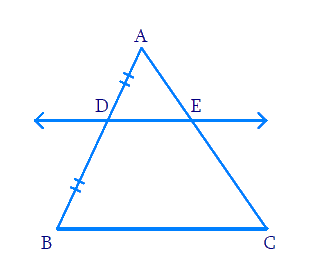# Ex.6.2 Q7 Triangles Solution - NCERT Maths Class 10

## Question

Using Theorem $$6.1$$, prove that a line drawn through the midpoint of one side of a triangle parallel to another side bisects the third side. (Recall that you have proved it in Class IX).Video Solution
Triangles
Ex 6.2 | Question 7

## Text Solution

Reasoning:

We know that theorem $$6.1$$ states that “If a line is drawn parallel to one side of a triangle to intersect the other two sides in distinct points, the other two sides are divided in the same ratio ($$BPT$$)”.

Steps:

In $$\Delta ABC$$$$, D$$ is the midpoint of $$AB$$

Therefore,

$AD = BD$

$\frac{AD}{BD}=1$

Now,

\begin{align}DE&||BC \\ \Rightarrow\frac{AE}{EC}&=\frac{AD}{BD}\,\,\,\text{[Theorem} \,6.1] \\ \Rightarrow \frac{AE}{EC}&=1 \end{align}

Hence $$,E$$ is the midpoint of $$AC.$$

Learn from the best math teachers and top your exams

• Live one on one classroom and doubt clearing
• Practice worksheets in and after class for conceptual clarity
• Personalized curriculum to keep up with school